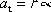a-sub-t = r times alpha

A point on a rotating object goes around in a circle. (The point "goes" while the object spins.) The radius r of that circle is the distance from the object's axis of rotation to the point. If the spin rate of the object is changing (meaning the object has some angular acceleration alpha) then the speed of the point is changing. The rate at which the point is speeding up along its circular path (a negative quantity if the point is actually slowing down) is called the tangential acceleration a-sub-t of the point. This equation states that the tangential acceleration of the point is equal to the radius of the circle times the angular acceleration of the spinning object.

Note: A point or an object that is going around in a circle always has centripetal acceleration. If the point or object is also speeding up or slowing down then it also has tangential acceleration.